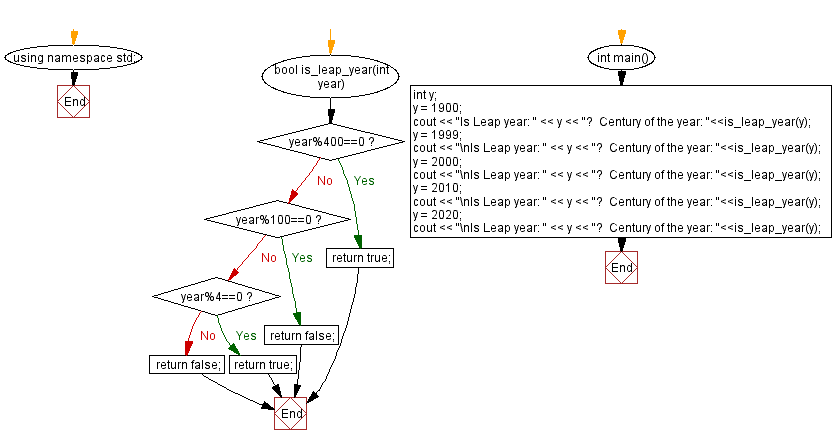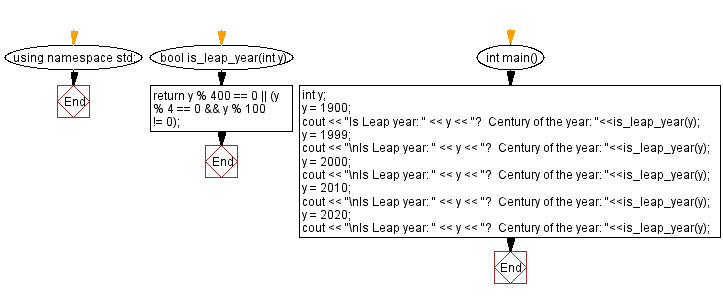﻿ C++ Exercises: Check whether a given year is a leap year not - w3resource

# C++ Exercises: Check whether a given year is a leap year not

## C++ Date: Exercise-4 with Solution

Write a C++ program to check whether a given year is a leap year or not.

Sample Solution-1:

C++ Code:

``````#include <iostream>

using namespace std;
bool is_leap_year(int year)
{
if(year%400==0)
return true;
if(year%100==0)
return false;
if(year%4==0)
return true;
return false;
}

int main()
{
int y;
y = 1900;
cout << "Is Leap year: " << y << "?  Century of the year: "<<is_leap_year(y);
y = 1999;
cout << "\nIs Leap year: " << y << "?  Century of the year: "<<is_leap_year(y);
y = 2000;
cout << "\nIs Leap year: " << y << "?  Century of the year: "<<is_leap_year(y);
y = 2010;
cout << "\nIs Leap year: " << y << "?  Century of the year: "<<is_leap_year(y);
y = 2020;
cout << "\nIs Leap year: " << y << "?  Century of the year: "<<is_leap_year(y);
}

``````

Sample Output:

```Is Leap year: 1900?  Century of the year: 0
Is Leap year: 1999?  Century of the year: 0
Is Leap year: 2000?  Century of the year: 1
Is Leap year: 2010?  Century of the year: 0
Is Leap year: 2020?  Century of the year: 1
```

Flowchart:Sample Solution-2:

C++ Code:

``````#include <iostream>

using namespace std;

bool is_leap_year(int y)
{
return y % 400 == 0 || (y % 4 == 0 && y % 100 != 0);
}

int main()
{
int y;
y = 1900;
cout << "Is Leap year: " << y << "?  Century of the year: "<<is_leap_year(y);
y = 1999;
cout << "\nIs Leap year: " << y << "?  Century of the year: "<<is_leap_year(y);
y = 2000;
cout << "\nIs Leap year: " << y << "?  Century of the year: "<<is_leap_year(y);
y = 2010;
cout << "\nIs Leap year: " << y << "?  Century of the year: "<<is_leap_year(y);
y = 2020;
cout << "\nIs Leap year: " << y << "?  Century of the year: "<<is_leap_year(y);
}
``````

Sample Output:

```Is Leap year: 1900?  Century of the year: 0
Is Leap year: 1999?  Century of the year: 0
Is Leap year: 2000?  Century of the year: 1
Is Leap year: 2010?  Century of the year: 0
Is Leap year: 2020?  Century of the year: 1
```

Flowchart:C++ Code Editor: